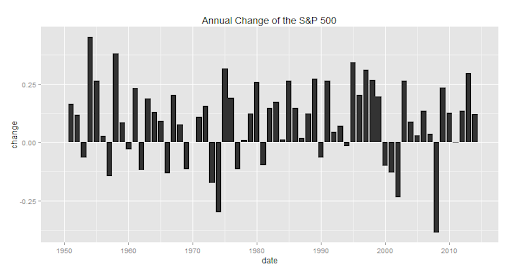## Monday, November 24, 2014

### Pipeline to Plot Annual % Change(thanks tradeblotter for pointing out error in code in first release)

Pipes in R make my life incredibly easy, and I think my code easier to read.   Note, there are a couple different flavors of pipes (see magrittr and pipeR).  For now, I choose pipeR.

`library(quantmod)library(pipeR)library(ggplot2)getSymbols("^GSPC",from="1900-01-01",auto.assign=F) %>>% #get S&P 500 from Yahoo!Finance  ( .[endpoints(.,"years"),4] ) %>>% #get end of year  ROC( type="discrete", n=1 ) %>>%   #get one year rate of change  na.fill(0) %>>%                    #fill first year with 0  (                                  #make data.frame    data.frame(      date = as.Date(format(index(.),"%Y-01-01"))      ,.    )  ) %>>%  structure(                         #hard way to do colnames()    names = c("date","change")  ) %>>%  ggplot(                            #start our plot pipe    aes( y= change, x=  date)  ) %>>%  + geom_bar( stat="identity" ) %>>% #choose bar  + labs( title = "Annual Change of the S&P 500" ) #give plot a title`

Or if we wanted to use the new pipeline syntax for the plot portion.

`library(quantmod)library(pipeR)library(ggplot2)getSymbols("^GSPC",from="1900-01-01",auto.assign=F) %>>% #get S&P 500 from Yahoo!Finance  ( .[endpoints(.,"years"),4] ) %>>% #get end of year  ROC( type="discrete", n=1 ) %>>%   #get one year rate of change  na.fill(0) %>>%                    #fill first year with 0  (                                  #make data.frame    data.frame(      date = as.Date(format(index(.),"%Y-01-01"))      ,.    )  ) %>>%  structure(                         #hard way to do colnames()    names = c("date","change")  ) %>>%  (    pipeline({      ggplot(., aes( y= change, x=  date) )      + geom_bar( stat="identity" )      + labs( title = "Annual Change of the S&P 500 (source: Yahoo! Finance)" )    })  )`